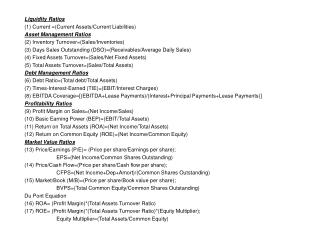DownloadDownload PresentationLiquidity Ratios (1) Current =(Current Assets/Current Liabilities) Asset Management Ratios

# Liquidity Ratios (1) Current =(Current Assets/Current Liabilities) Asset Management Ratios

Télécharger la présentation## Liquidity Ratios (1) Current =(Current Assets/Current Liabilities) Asset Management Ratios

- - - - - - - - - - - - - - - - - - - - - - - - - - - E N D - - - - - - - - - - - - - - - - - - - - - - - - - - -
##### Presentation Transcript

1. Liquidity Ratios (1) Current =(Current Assets/Current Liabilities) Asset Management Ratios (2) Inventory Turnover=(Sales/Inventories) (3) Days Sales Outstanding (DSO)=(Receivables/Average Daily Sales) (4) Fixed Assets Turnover=(Sales/Net Fixed Assets) (5) Total Assets Turnover=(Sales/Total Assets) Debt Management Ratios (6) Debt Ratio=(Total debt/Total Assets) (7) Times-Interest-Earned (TIE)=(EBIT/Interest Charges) (8) EBITDA Coverage=[(EBITDA+Lease Payments)/(Interest+Principal Payments+Lease Payments)] Profitability Ratios (9) Profit Margin on Sales=(Net Income/Sales) (10) Basic Earning Power (BEP)=(EBIT/Total Assets) (11) Return on Total Assets (ROA)=(Net Income/Total Assets) (12) Return on Common Equity (ROE)=(Net Income/Common Equity) Market Value Ratios (13) Price/Earnings (P/E)= (Price per share/Earnings per share); EPS=(Net Income/Common Shares Outstanding) (14) Price/Cash Flow=(Price per share/Cash flow per share); CFPS=(Net Income+Dep+Amort)/(Common Shares Outstanding) (15) Market/Book (M/B)=(Price per share/Book value per share); BVPS=(Total Common Equity/Common Shares Outstanding) Du Pont Equation (16) ROA= (Profit Margin)*(Total Assets Turnover Ratio) (17) ROE= (Profit Margin)*(Total Assets Turnover Ratio)*(Equity Multiplier); Equity Multiplier=(Total Assets/Common Equity)